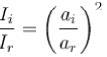Courses

# Waves MCQ Level - 2

## 10 Questions MCQ Test Topic wise Tests for IIT JAM Physics | Waves MCQ Level - 2

Description
This mock test of Waves MCQ Level - 2 for IIT JAM helps you for every IIT JAM entrance exam. This contains 10 Multiple Choice Questions for IIT JAM Waves MCQ Level - 2 (mcq) to study with solutions a complete question bank. The solved questions answers in this Waves MCQ Level - 2 quiz give you a good mix of easy questions and tough questions. IIT JAM students definitely take this Waves MCQ Level - 2 exercise for a better result in the exam. You can find other Waves MCQ Level - 2 extra questions, long questions & short questions for IIT JAM on EduRev as well by searching above.
QUESTION: 1

### When a source of sound of frequency crosses a stationary observer with a speed v, ( « speed of sound v), the apparent change in frequency Δf is given by :

Solution: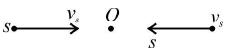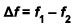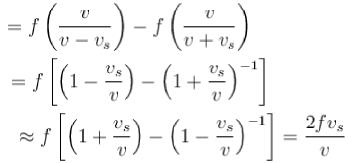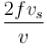QUESTION: 2

### In a stationary wave that forms as a result of reflection of wave from an obstacle the ratio of the amplitude at an antinode to the amplitude at node is n. The fraction of energy reflected is :

Solution:

Let ai, and ar be the amplitudes of incident and reflected waves. Then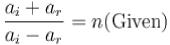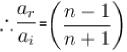∴ Fraction of energy reflected is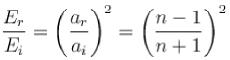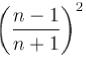QUESTION: 3

### The displacement y of a particle executing periodic motion is given by :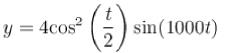The expression may be consider to be a result of the superposition of independent harmonic motions :

Solution:

The given equation can be written as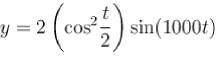y = 2(cost + 1) sin(1000t)
= 2cost sin 1000t + 2sin(1000t)
= sin(1001t) + sin(999t) + 2sin(1000t)
i.e. the given expression is a small of superposition of three independent harmonic motions of angular frequencies 999,1000 and 1001 rad/sec.

QUESTION: 4

The displacement of particles in a string stretched in the x-direction is represented by y.  Among the following expressions for y, those describing wave motion are :

Solution:

Only first equation is the equation of standing wave. The condition for a function x and t to represent a wave is :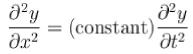Only first equation satisfies the condition.
The correct answer is: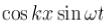QUESTION: 5

A transverse wave is described by the equation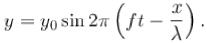The maximum particle velocity is equal to four times the wave velocity if :

Solution:

Wave velocity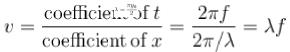Maximum particle velocity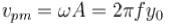Given,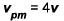or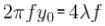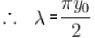The correct answer is: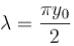QUESTION: 6

A transverse wave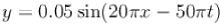in metres, is propagating along +ve X- axis on a string. A light insect starts crawling on the string with the velocity of 5 cm/s at t = 0 along the +ve X- axis from a point where x = 5 cm . After 5 s  the difference in the phase of its position is equal to :

Solution:

At t = 0, x = 5 cm, initial phase of insect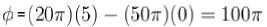After 5s insect will more a distance s = vt = 25 cm
So, it will beat x = 30 cm .
At this instant,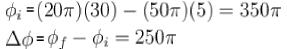The correct answer is: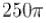QUESTION: 7

Speed of sound wave is v. If a reflector moves towards a stationary source emitting waves of frequency f with speed u, the wavelength of reflected wave will be :

Solution:

Apparent frequency for reflector (will act as an observer) would be :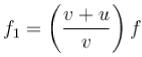where f = actual frequency of source.
After being reflected the apparent frequency will further change and the reflector will now behave as a source. The apparent frequency will now become :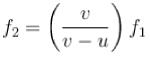Substituting value of f1, we get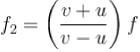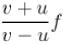QUESTION: 8

A train is moving with a constant speed along a circular track. The engine o f the train emits a sound of frequency f. The frequency heard by the guard at rear end of the train :

Solution:

Let v be the speed of sound and u the speed of train.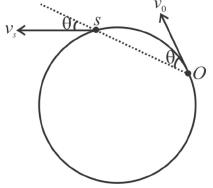Then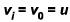and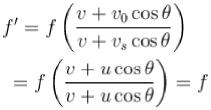The correct answer is: is equal to f

QUESTION: 9

A standing wave is maintained in a homogeneous string of cross-sectional area a and density p. It is formed by superposition of two waves travelling in opposite directions given by the equation.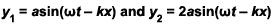The total mechanical energy confined between the sections corresponding to the adjacent antinodes is :

Solution:

Distance between two adjacent antinodes is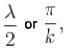Volume of string between two adjacent antinodes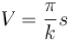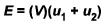Here, u is the energy density (energy per unit volume) which is equal to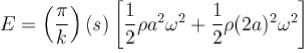The correct answer is :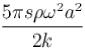QUESTION: 10

A travelling wave is partialy reflected partialy and partly transmitted from a rigid boundary. Let av ar and at be the amplitudes of incident wave, reflected wave and transmitted wave and li, Ir and lt be the corresponding intensities. Then choose the correct alternative:

Solution: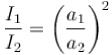only when other properties like density of medium, speed of wave etc, remains the same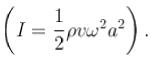In incident and reflected waves medium is same. Therefore, other properties are same. Hence,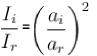The correct answer is: